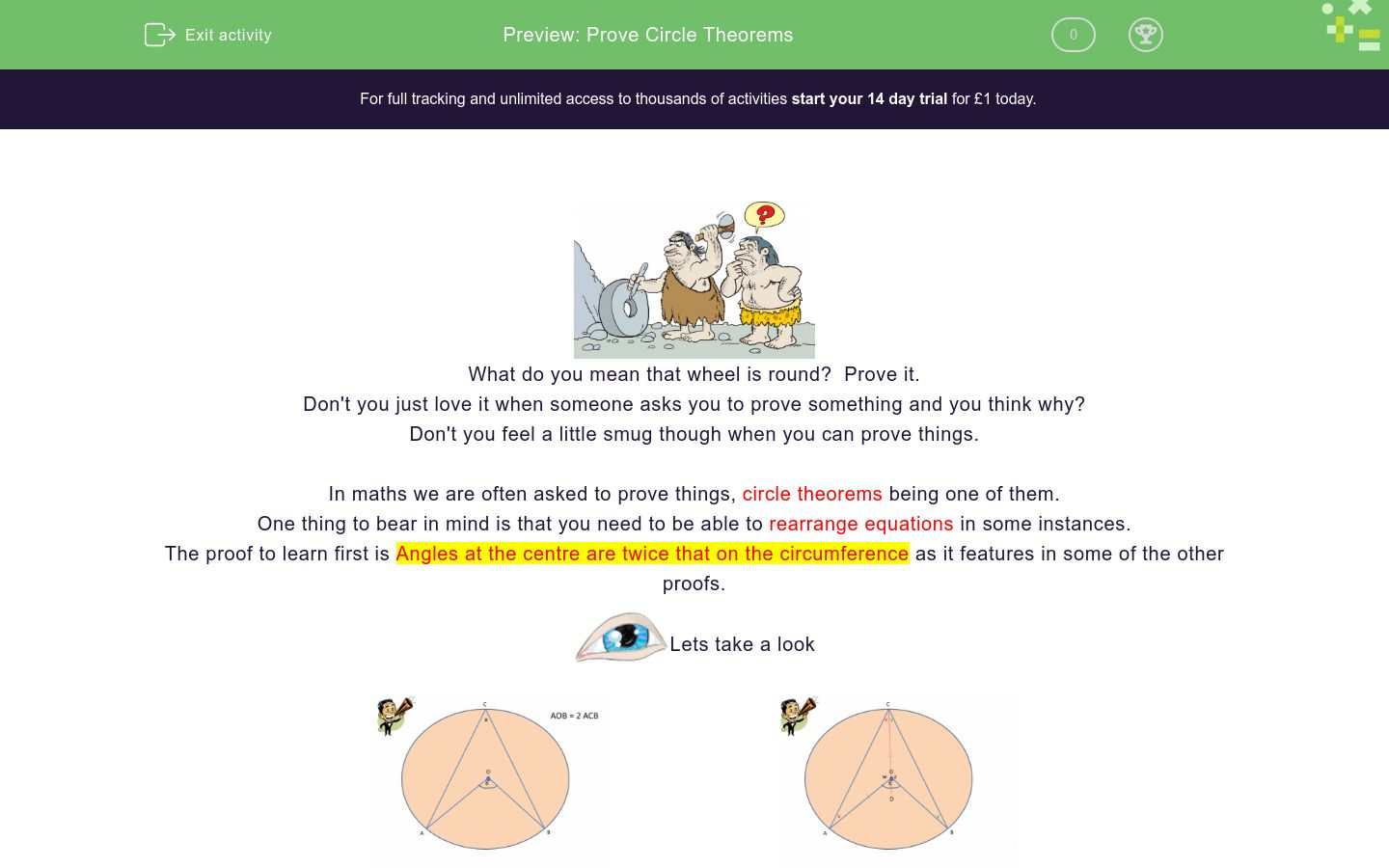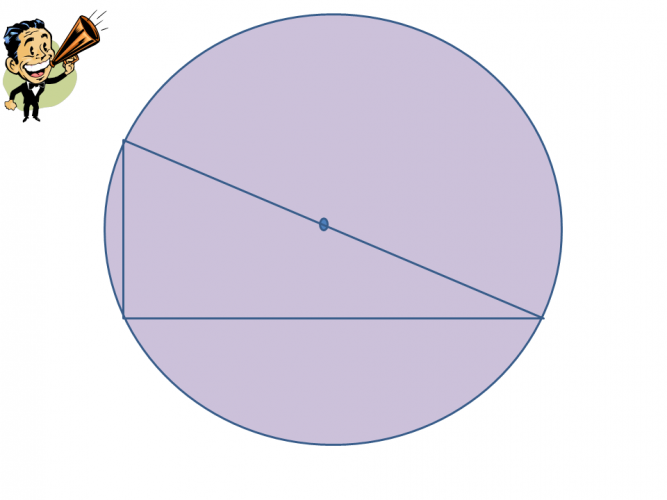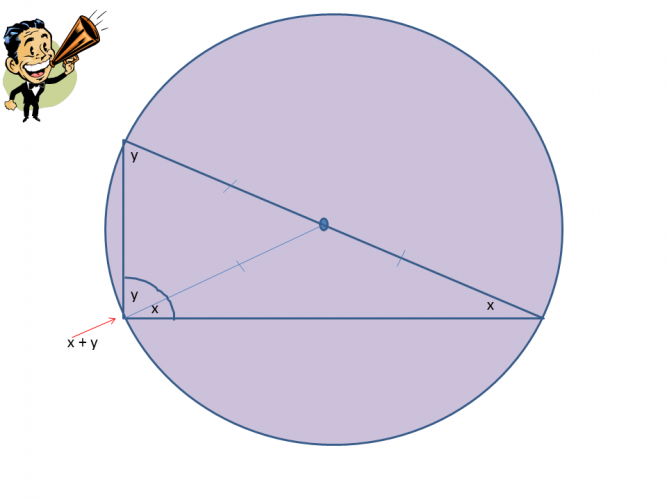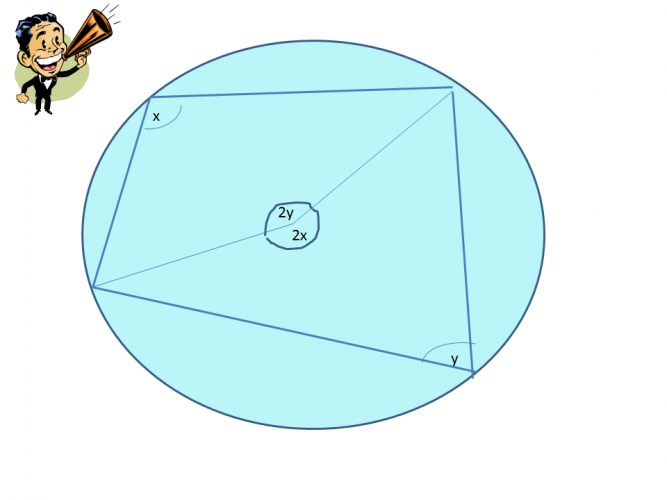# Prove Circle Theorems

In this worksheet, students will learn to prove the theorems they have been working with.Key stage:  KS 4

GCSE Subjects:   Maths

GCSE Boards:   AQA, Eduqas, OCR, Pearson Edexcel

Curriculum topic:   Geometry and Measures, Basic Geometry

Curriculum subtopic:   Properties and Constructions, Circles

Difficulty level:### QUESTION 1 of 10What do you mean that wheel is round?  Prove it.

Don't you just love it when someone asks you to prove something and you think why?

Don't you feel a little smug though when you can prove things.

In maths we are often asked to prove things, circle theorems being one of them.

One thing to bear in mind is that you need to be able to rearrange equations in some instances.

The proof to learn first is Angles at the centre are twice that on the circumference as it features in some of the other proofs.Lets take a lookFirst of all draw a line from C through the centre.  You can see that two isosceles triangles have been formed.

The triangle on the left has been labelled as w, x and x and the triangle on the right has been labelled as z, y and y.

We know

w = 180° - 2x   and z = 180° - 2y

Angles around a point add up to 360°

Therefore w + z + b = 360°.

Now substitute in w and z

180 - 2x + 180 - 2y + b = 360°

Simplify

360° -  2x - 2y +  b  =  360°

Rearrange to get b on its own

-2x -2y + b = 0

b = 2x + 2y

B = 2(x+y)

We re labelled the diagram at the beginning with x and y in place of a. B is at the centre and we know that the angle at the centre is twice the angle on the circumference.  The proof is all there.

Angle from the diameter (also known as angles in a semi circle)Draw a line from the centre of the circle to the right angle.  This has formed a radius. label each side of the radius x and y.

We know

x + x + y + y = 180°

Simplify

2x + 2y = 180°

Simplify further/ Cancel down

x + y = 90° (The angles at the right angle)

Angles in the same segment are equalIf you draw in a chord and two radii from the centre, you can see angles at the centre are twice that on the circumference.

Whatever angle is at the centre the angle at the circumference will be half.  So angle a and b must be the same.If you draw in two radii from the centre you can see that the angles in the centre rule creeps in.

We know

2x + 2y = 360°

Simplify/Cancel down

x + y = 180° (opposites sides of the quadrilateral)

Alternate segment proofDraw radii in from the centre

Form two right angles triangles.

We know

a + x = 90° (Angles that meet at a tangent)

90 + x + y = 180°

Simplify

x + y = 90° remember a + x  also equals 90°, therefore a = y (whatever x is a and y will be the same)

Look at angle B.

We know that angle B is half that of that of the angles in the centre 2y or to be more precise that angle at the centre is twice that on the circumference. (angles at centre theorem)

Now double b to get 2b = 2y.

Halve the equations gives us b = y

We already have found that a = y and can also now see that b = y. Hence proving the alternate segment theorem.

Tangents meet a radius at 90°:Use your imagination for this one.

Draw a line in from the centre to another point on the tangent, as shown in the diagram above.

Suppose OB is not perpendicular to line AD.

Suppose OC is perpendicular to line AD

This would mean that angle OCB = 90° and OBC would be acute.

The greater side is opposite the greatest angle, using this scenario we can see that this is not the case.

OB = OE and OE > OC which is impossible.

Therefore it is only the line that meets the tangent at the circumference is perpendicular proving that OB is perpendicular to AD and therefore 90°.

Give the following a go.From the list below what theorems are used to prove that opposite angles in a cyclic quadrilateral add up to 180°

Angle at the centre is twice that on the circumference

Angles in a triangle add up to 180°

Angles around a point add up to 360°

Opposite angles add up to 90°Prove that  the angle subtended (made) from the diameter is equal to 90°.What other circle theorem can help you prove that angles in the same segment are equal?

Angles at the centre are twice that of on the circumference

Opposite angles add up to 180°

Angles that meet at the circumference at a tangent are 90°

Angles around a point add up to 360°a + x = 90° and angles at the centre are twice that on the circumference.

This is part of the proof of which circle theorem?

Angles at the centre are twice that of on the circumference

Opposite angles add up to 180°

Angles that meet at the circumference at a tangent are 90°

Angles around a point add up to 360°What two numbers are important in helping prove angles at the centre are twice that on the circumference?Which is the correct proof for showing that angles at the centre are twice that on the circumference.

 A w = 180° - 2x   and z = 180° - 2y  w + z + b = 360°. 180 - 2x + 180 - 2y + b = 360° 360° -  2x - 2y +  b  =  360° -2x -2y + b = 0 b = 2x + 2y B = 2(x+y) B w = 360° - 2x   and z = 360° - 2y  w + z + b = 360°. 360 - 2x + 360 - 2y + b = 180° 360° -  2x - 2y +  b  =  360° -2x -2y + b = 0 b = 2x + 2y B = 2(x+y)What can you do to help prove that angles at a tangent meet the circumference at 90°

Draw in radii to help identify the right angles

Subtract 180° from 360°

State that angles around a point add up to 360°

Use the angle at the centre is twice that on the circumference.

• Question 1From the list below what theorems are used to prove that opposite angles in a cyclic quadrilateral add up to 180°

Angle at the centre is twice that on the circumference
Angles around a point add up to 360°
EDDIE SAYS
Did you have a go at drawing the shape out and trying to prove the theorem? Always have a go at trying to prove the theorem as the more you practice the easier they seem. You should have drawn two radii from the centre and then you can apply 'angles at the centre are twice that on the circumference theorem' to help with the proof. 2x + 2y = 360° simplified becomes x + y = 180° The theorem is that opposite angles in a cyclic quadrilateral add up to 180°
• Question 2Prove that  the angle subtended (made) from the diameter is equal to 90°.

x + x + y + y = 180°, 2x + 2y = 180°, x + y = 90°
EDDIE SAYS
x + x + y + y = 180° 2x + 2y = 180° x + y = 90° (The angles at the right angle) If you draw a line in from the centre to the right angle you create two triangles. Label the angles x and y and you should come up with the above.
• Question 3What other circle theorem can help you prove that angles in the same segment are equal?

Angles at the centre are twice that of on the circumference
EDDIE SAYS
Did you get this one? You will find that this one creeps in to some of the other proofs. I think that one is the most time consuming to prove but is worth it as it helps in so many other areas.
• Question 4a + x = 90° and angles at the centre are twice that on the circumference.

This is part of the proof of which circle theorem?

EDDIE SAYS
Draw radii in from the centre to help see the proof as two right angles are formed. a + x = 90° (Angles that meet at a tangent) 90 + x + y = 180° Simplify x + y = 90° remember a + x also equals 90°, therefore a = y (whatever x is a and y will be the same) 2b = 2y. b = y We already have found that a = y and can also now see that b = y. Hence proving the alternate segment theorem.
• Question 5What two numbers are important in helping prove angles at the centre are twice that on the circumference?

180 and 360
EDDIE SAYS
We use 180° because the good old triangle is formed when trying to prove this theorem. 360° because angles around a point add up to 360°
• Question 6Which is the correct proof for showing that angles at the centre are twice that on the circumference.

 A w = 180° - 2x   and z = 180° - 2y  w + z + b = 360°. 180 - 2x + 180 - 2y + b = 360° 360° -  2x - 2y +  b  =  360° -2x -2y + b = 0 b = 2x + 2y B = 2(x+y) B w = 360° - 2x   and z = 360° - 2y  w + z + b = 360°. 360 - 2x + 360 - 2y + b = 180° 360° -  2x - 2y +  b  =  360° -2x -2y + b = 0 b = 2x + 2y B = 2(x+y)

A
EDDIE SAYS
Did you try and write out the proof for yourself? I hope so as this is the only way to learn it. Keep practicing if you need to.
• Question 7What can you do to help prove that angles at a tangent meet the circumference at 90°

Draw in radii to help identify the right angles
EDDIE SAYS
Always draw on the diagram, thats what it is there for. Draw in the right angle symbols two and use the word perpendicular in your answer which means at right angles to.
---- OR ----

Sign up for a £1 trial so you can track and measure your child's progress on this activity.

### What is EdPlace?

We're your National Curriculum aligned online education content provider helping each child succeed in English, maths and science from year 1 to GCSE. With an EdPlace account you’ll be able to track and measure progress, helping each child achieve their best. We build confidence and attainment by personalising each child’s learning at a level that suits them.

Get started